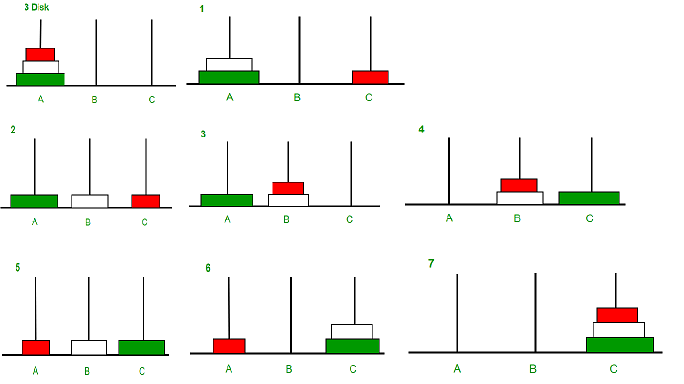# Tower of Hanoi

## Exercise

Tower of Hanoi is a mathematical puzzle where we have three rods and n disks. The objective of the puzzle is to move all disks on one rod to another rod, obeying the following simple rules:

• Only one disk can be moved at a time.
• Each move consists of taking the upper disk from one of the rods and placing it on top of another rod i.e. a disk can only be moved if it is the uppermost disk on a rod.
• No disk may be placed on top of a smaller disk.

In addition to moving the disks to another rod the following requirements have to be fulfilled:

• Before executing the algorithm read the number of disks from the console
• Each disk is identified by it's size which is stored as an integer value
• After each step (moving one disk to another rod) print out the current state of the three rods by printing out the sizes of the disks for each rod
• When all disks are located on another rod print out the number of steps which were performed

## Example Input / Output

The following console log shows an example of the different events which took place while solving the puzzle:

``````Type in the number of disks: 3

Solving the puzzle:

Step 0:
Rod A: 3 2 1
Rod B: -
Rod C: -

Step 1:
Rod A: 3 2
Rod B: -
Rod C: 1

Step 2:
Rod A: 3
Rod B: 2
Rod C: 1

...

Step 7:
Rod A: -
Rod B: -
Rod C: 3 2 1

Puzzle solved in 7 steps!
``````

## Example for 3 disksSource: